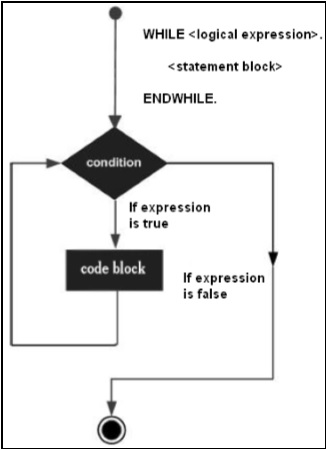# SAP ABAP - While Loop

A WHILE loop statement repeatedly executes a target statement as long as a given condition is true.

The general format for the WHILE command is as follows −

```WHILE <logical expression>

<statement block>.

ENDWHILE.
```

The statement block may be a single statement or a block of statements.

The WHILE loop executes the statements enclosed by the WHILE and ENDWHILE commands until the logical expression becomes false.

## Flow DiagramThe WHILE command is preferable while considering the performance of programs. The loop continues until the logical statement is found to be untrue and exits the loop if a false statement is found, and the first statement after the WHILE loop is executed.

## Example

```REPORT YS_SEP_15.

DATA: a type i.

a = 0.

WHILE a <> 8.

Write: / 'This is the line:', a.
a = a + 1.

ENDWHILE.
```

The above code produces the following output −

```This is the line: 0
This is the line: 1
This is the line: 2
This is the line: 3
This is the line: 4
This is the line: 5
This is the line: 6
This is the line: 7
```
sap_abap_loop_control.htm